# RBSE Maths Class 8 Chapter 12: Important Questions and Solutions

RBSE Maths Chapter 12 – Linear Graph Class 8 Important questions and solutions can be used as study material to face the board exams. All the important questions from previous years, additional questions and answers covering all the concepts of Chapter 12 contain step by step answers, along with explanations. All these important questions are authored according to the new pattern prescribed by the RBSE. Students can also get the syllabus and textbooks on RBSE Class 8 solutions.

Chapter 12 of the RBSE Class 8 Maths will help the students to solve problems related to the cartesian system, coordinates, representation of some real relationship through a graph.

### RBSE Maths Chapter 12: Exercise 12.1 Textbook Important Questions and Solutions

Question 1: Fill in the blanks using the graph given below.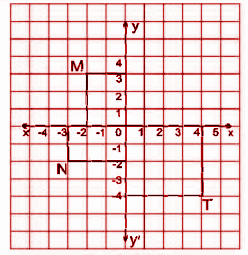(i) Distance from the x-axis of a point M is _____ unit.

(ii) Distance from the y-axis of a point M is _____ unit.

(iii) Distance from the y-axis of a point N is _____ unit.

(iv) Point T is plotted on quadrant _____.

(v) Distance from the x-axis of a point T is _____ unit.

Solution:

(i) 3 (ii) 2 (iii) 3 (iv) fourth (v) 4

Question 2: Fill in the blanks using the graph given below.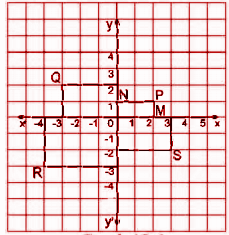(i) Abscissa _____ and ordinate _____ are of point P. Therefore, the coordinates of point P are _____.

(ii) Abscissa _____ and ordinate _____ are of point Q. Therefore, the coordinates of point Q are _____.

(iii) x-coordinate _____ and y-coordinate _____ are of point R. Therefore, the coordinates of point R are _____.

(iv) x-coordinate _____ and y-coordinate _____ are of point S. Therefore, the coordinates of point S are _____.

Solution:

(i) 2, 1, (2,1)

(ii) – 3, 2, (- 3, 2)

(iii) -4, -3, (- 4,- 3)

(iv) 3,- 2, (3, – 2)

Question 3: Plot the following points and check whether they lie on a straight line.

(i) A (1, 1); B (1, 2); C (1,3); D (1, 4).

(ii) K (1, 3); L (5,3); M (5, 5); N (1, 5).

(iii) P (2, 6); Q (5, 5); Y (5, 3); Z (6, 3).

Solution:

[i]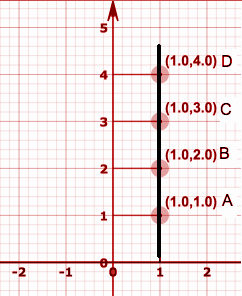All the points A, B, C and D lie on a straight line and are collinear.

[ii]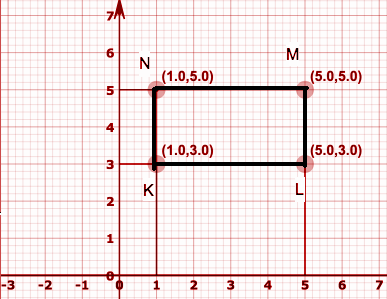All the points K, L, M and N don’t lie on a straight line and hence are not collinear.

[iii]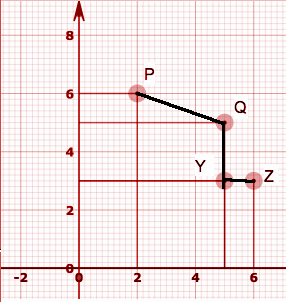All the points P, Q, Y and Z don’t lie on a straight line and hence are not collinear.

Question 4: Draw the following graph on a graph paper and answer the questions given below.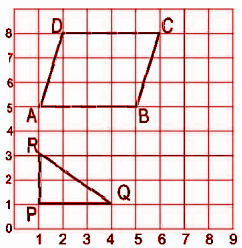[i] What are the coordinates of parallelogram ABCD? Also, find the length of the sides AB and DC.

[ii] Find the coordinates of the vertices of the triangle PQR. Measure the length of the base PQ.

Solution:

[i] The coordinates of the parallelogram are as follows:

A (1, 5), B (5, 5), C (6, 8) and D (2, 8).

The length of AB = 4 units.

The length of DC = 4 units.

[ii] The coordinates of the vertices of the triangle PQR are:

P (1, 1), Q (4, 1) and R (1, 3).

The length of the base PQ = 3 units.

Question 5: State whether the following statements are true or false.

(i) The location of a point on a graph paper is represented by the number pair.

(ii) The linear graph shows the change in data with respect to time-interval.

(iii) The point having x-coordinate zero and y-coordinate non-zero is located on the y-axis.

(iv) The point having y-coordinate zero and x-coordinate 5, is located on the y-axis.

(v) Coordinates of origin are (1,1).

Solution:

(i) True (ii) True (iii) True (iv) False (v) False.

### RBSE Maths Chapter 12: Exercise 12.2 Textbook Important Questions and Solutions

Question 1: The length of a side of

[i] an equilateral triangle and

[ii] square is ‘x’ cm. Find their perimeter and plot a graph between the perimeter and its length.

Solution:

The perimeter of an equilateral triangle = 3 * [length of one side] = 3x

The table below gives the value of the perimeter for the corresponding side.

 Length of side [x] 1 2 3 4 5 6 Perimeter [P] 3 6 9 12 15 18
[i]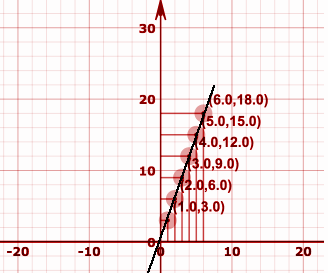Scale:

x-axis = 1unit = 10cm

y-axis = 1unit = 10cm

[ii] The perimeter of a square = 4 * [length of one side] = 4x

The table below gives the perimeter for the corresponding length of the side.

 Length of side [x] 1 2 3 4 5 6 Perimeter [P] 4 8 12 16 20 24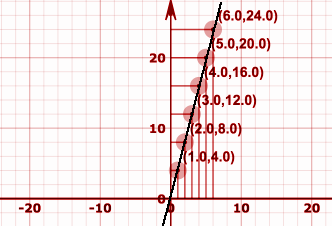Scale:

x-axis = 1unit = 10cm

y-axis = 1unit = 10cm

Question 2: The length of a rectangle is double its breadth. Plot a graph of the area and breadth of the rectangle.

Solution:

According to the question, length = 2 * length.

Area of a rectangle = length * breadth = length * 2 * length.

If the length of a rectangle is ‘x’ units, then the area of a rectangle is 2x2.

The table below gives the value of the area for the corresponding breadth.

 The breadth of side [x] 1 2 3 4 5 Area [A] 2 8 18 32 50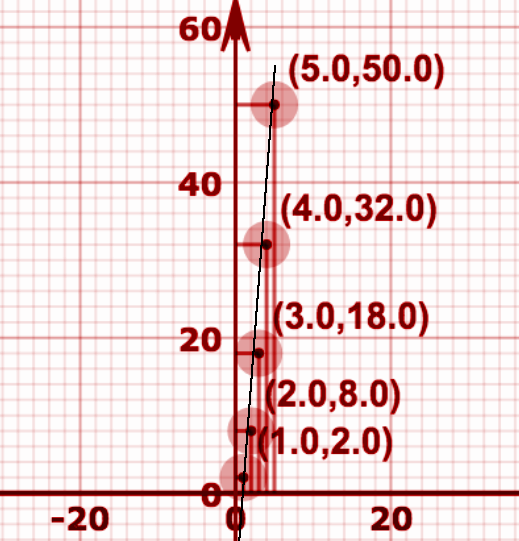Scale:

x-axis = 1unit = 20cm

y-axis = 1unit = 20cm

Question 3: According to the table, draw a graph between time and simple interest.

 Time 1st year 2nd year 3rd year 4th year Simple Interest Rs. 60 Rs. 120 Rs. 180 Rs. 240

Solution: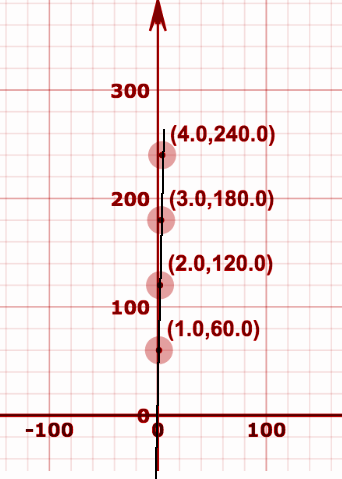Scale:

x-axis = 1unit = 100cm

y-axis = 1unit = 100cm

Question 4: Plot a graph of the relation between time and distance.

 Time 2 4 6 8 Distance 10 20 30 40

Solution: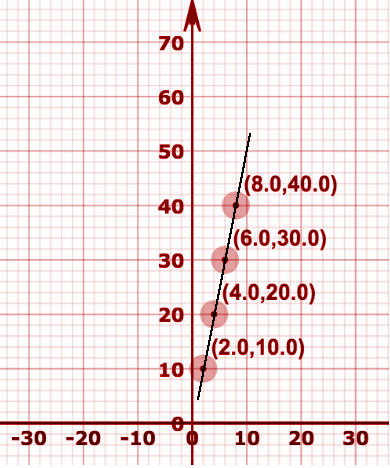Scale:

x-axis = 1unit = 10cm

y-axis = 1unit = 10cm

Question 5: Plot the graph for the values given in the table and explain why this graph passes through the origin?

 Fixed Deposit [in Rs.] 1000 2000 3000 4000 5000 Simple Interest [in Rs.] 80 160 240 320 400

Solution: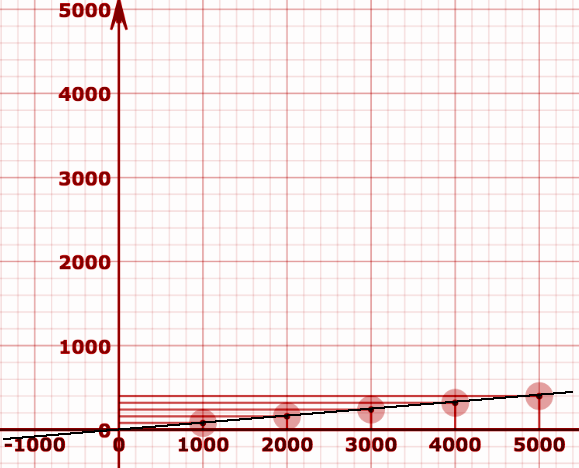Scale:

x-axis = 1unit = 1000cm

y-axis = 1unit = 1000cm

### RBSE Maths Chapter 12: Additional Questions and Solutions

Question 1: Looking at the graph given below, choose the appropriate alphabets for the location of the following points.

(i) (2, 1)

(ii) (0, 5)

(iii) (2, 0)

(iv) coordinates of point A

(v) coordinates of point F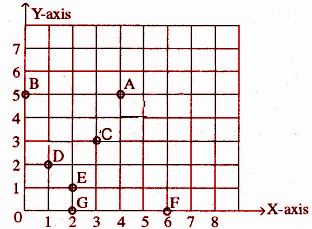Solution:

(i) Point E

(ii) Point B

(iii) Point G

(iv) (4, 5)

(v) (6, 0)

Question 2: Plot the following coordinates and check whether they lie on a straight line.

[i] P (0, 2), Q (0, 5), R (0, 6), S (0, 3)

[ii] A (1, 1), B (1, 2), C (1, 3), D (1, 4)

[iii] K (1, 3), L (2, 3), M (3, 3), N (4, 3)

[iv] W (2, 6), X (3, 5), Y (5, 3), Z (6, 2)

Solution:

[i] P (0, 2), Q (0, 5), R (0, 6), S (0, 3)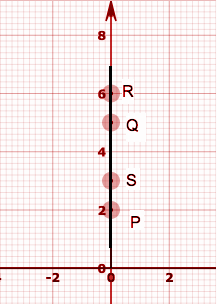The given points lie on a straight line.

[ii] A (1, 1), B (1, 2), C (1, 3), D (1, 4)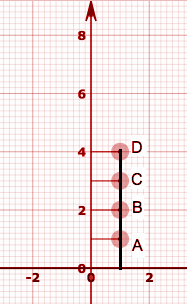The given points lie on a straight line.

[iii] K (1, 3), L (2, 3), M (3, 3), N (4, 3)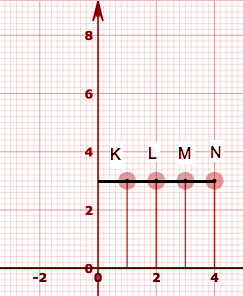The given points lie on a straight line.

[iv] W (2, 6), X (3, 5), Y (5, 3), Z (6, 2)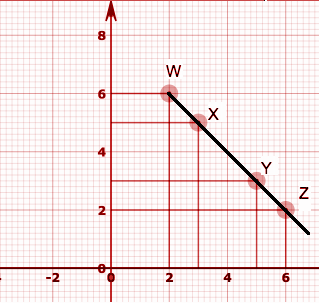The given points lie on a straight line.